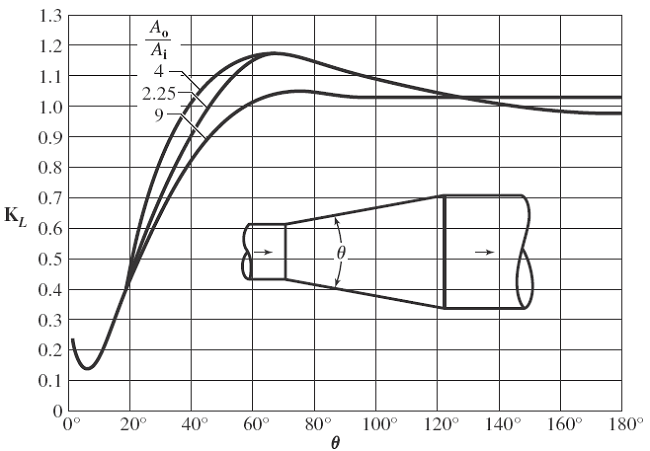Related Resources: calculators

### Pipe Flow Gradual Size Enlargement Formula and Calculator

Hydraulic and Pneumatic Knowledge

Pipe Flow with Gradual Size Enlargement Formula and Calculator:

Gradual enlargement causes less energy loss than sudden enlargement because it causes less turbulence. Diffusers are gradual enlargement device. Velocity decreases while pressure increases. Gradual enlargements generally have a conical section that joins two different pipe diameters. The divergence angle is the included angle in the cone. The following two equations give approximations of head loss in a gradual enlargement. KL is the Gibson loss coefficient (see Figure 1).

KD is the resistance coefficient (see Fig. 2). Both depend on the ratio of areas and the angle of divergence. The formulas to calculate this loss ignore the effects of friction inside the conical section. If the enlargement is very gradual, the effects of friction can become significant. Therefore, an included angle of 6° or more is recommended in the conical section to mitigate the effects of friction. For area ratios up to 6:1 with angles of divergence of 40° or more, head loss may be less with a sudden expansion than with a gradual one. These two effects are clearest on the graph of KL (Figure 1).

hl = KD ( vi2 / ( 2 g ) ) Eq 1

hl = KL [ (vi2 - vo2 ) / 2 g ] Eq 2

where:

vi = velocity entering the enlargement,
vo = velocity leaving the enlargement,
hl = head loss in units of length,
KD = resistance coefficient see figure 2,
KL = Gibson loss coefficient see figure 1,
g = gravity (32.174 ft/sec2),

Head Loss Coefficient KL, Conical Diffuser
Table 1 (Click on image to enlarge)Head Loss Coefficient KD, Conical Diffuser
Table 2Related:

Source: Machinery Handbook, 28th Edition